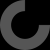MakerBot Print is our newest print-prepration software, which supports native CAD files and STL assemblies,
allows you to interact with all your printers via the Cloud, and many other exciting new features.

# Report as inappropriate

Well it's not entirely your fault... If you look closely into the library you may notice that the coordinates for the "F" and "G" points, which define the inner wall, are set to "d-4*k/2 * ..some_function.." which basically means that it subtracts 4 times the thread_pitch from the diameter. In your case (12-4*4).. results in a negative value.
I've tried to set the x,y coordinates of these points to '0' so that the nasty hole in the middle would be closed all together, sadly this too results in errors...
You can however set the width of the hole to a fixed size, change the lines for these two points by deleting the proportional factor "d-4k/2". Those lines should then read:
/* F */ for(i=[0:n]) [cosinus(n,i),sinus(n,i),height(k,n,i,-0.5)],
/* G */ for(i=[n*(m-1):n*m]) [cosinus(n,i),sinus(n,i),height(k,n,i,+0.5)],
The hole in the center should now always have the same diameter of 2mm (to avoid issues the cylinder in the "union" should be bigger than 2)

Another thing, in your module you cut away the top and bottom of the thread. When you later subtract the module from the bigger cylinder you run into trouble. The object you subtract from the other one should always overlap at least tiny bit.
Your example then can be written much easier:

\$fn=60;
module center(){
union(){
translate([0,0,-5])
cylinder(h=35,d=2.5,center=false);
translate([0,0,-5])
}
}
difference(){
cylinder(h=25,d=25,center=false);
center();
}

or without the 'module' even just:
\$fn=60;
difference(){
cylinder(h=25,d=25,center=false);
translate([0,0,-5]) s_thread(12, 4, 35);
translate([0,0,-5]) cylinder(h=35,d=2.5,center=false);
}